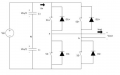# Single Phase Full Bridge Inverter

#### nath_h

Joined Sep 26, 2015
2
Hey Guys,

I am designing and building a single phase full bridge inverter that is either voltage/current controlled. I have essentially got the simulations of the control and inverter working as they should. However I am onto the specification of the components i.e. MOSFETs, drivers, optocouplers etc.

The inverter ratings are as follows: 1 kW, Vin = 48 Vdc, Iin =~20.9 A

Here's essentially what the circuit looks like without the control feedback, pure R load and output LC filter:I have simulated using SPWM, unipolar switching the FETs at 21 kHz frequency, getting an output of approx 50 Hz.

For the component sizing and selection I am using a tolerance of +20% on the maximum values of current and voltage thus;
Vmax = 1.2(48) = 57.6 V
Imax = 1.2(21) = 25.2 A

What I am asking about is the FET ratings that I should be using, and to verify if they're going to work with what I want. I have been looking and researching FETS and came up with this MOSFET: http://docs-asia.electrocomponents.com/webdocs/1300/0900766b813009ca.pdf

It has a maximum Drain-Source voltage of 60 V, and maximum continuous current of 50 A, which both are acceptable in retrospect with the maximum ratings of the inverter. It has a gate source voltage of +- 20 V, which the FET driver will need to supply. However I am wondering on how to go about calculating/verifying that the FET can switch at the required frequency / time intervals?

If I switch at 21 kHz, t = 1/f = 1/21x10^3 = 47.62 uS

This means that the FET switches every 47.62 micro seconds. From Vishay IRFZ48 datasheet summing the turn on delay time, rise time, fall time and turn off delay time:

t1 = 8.1+250+210+250 = 718.1 nS

So I'm assuming that this means that the Vishay IRFZ48 takes 718.1 nS to reach steady state every time it switches of which it will then be on for the pulse width duration, but from here I'm kind of lost. Also from what I gathered, these times are at a Vgs (gate signal voltage) of 10 V, not the specified 20 V, what effect would this have on the times?

Any help / guidance would be very much appreciated, thank you!

#### Dodgydave

Joined Jun 22, 2012
10,829
How can you switch at 21khz and get an output of 50hz???